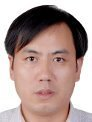Electron. J. Differential Equations, Vol. 2018 (2018), No. 47, pp. 1-14.

### Properties on measure pseudo almost automorphic functions and applications to fractional differential equations in Banach spaces Yong-Kui Chang, Tian-Wei Feng

Abstract:
In this article, we establish some new composition theorems on measure pseudo almost automorphic functions via measure theory. The obtained compositions theorems generalize those established under the well-known Lipschitz conditions or the classical uniformly continuous conditions. Then using the theories of resolvent operators and fixed point theorem, we investigate the existence and uniqueness of measure pseudo almost automorphic solutions to a fractional differential equation in Banach spaces.

Submitted January 3, 2018. Published February 15, 2018.
Math Subject Classifications: 34C27, 43A60, 34A08.
Key Words: Composition theorems; measure pseudo almost automorphic function; fractional differential equation.

Show me the PDF file (274 KB), TEX file for this article.Yong-Kui Chang School of Mathematics and Statistics Xidian University Xi'an 710071, China email: lzchangyk@163.com Tian-Wei Feng Department of Mathematics Lanzhou Jiaotong University Lanzhou 730070, China email: 502772153@qq.com